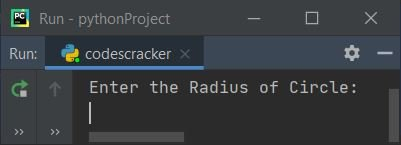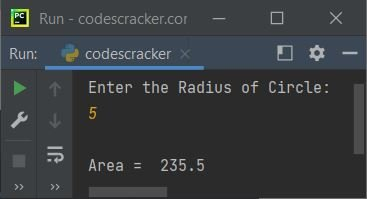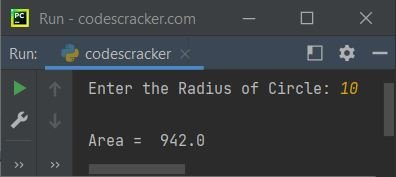# Python Program to Find Area of Circle

In this article, we've created some programs in Python to find and print area of a circle using its radius value entered by user at run-time. Here are the list of programs:

• Find Area of Circle without Function
• Find Area of Circle using User-defined Function
• Using Class

### Formula to Find Area of Circle

To find area of a circle with given value of radius, use following formula:

```a = 3πr2
= 3*3.14*r*r```

Here a indicates to area value, r indicates to radius value of circle.

Note - The value of π is 3.14.

## Find Area of Circle

This program receives radius of circle as input from user at run-time, then find and prints its area. The question is write a Python program to compute area of a circle. Here is its answer:

```print("Enter the Radius of Circle: ")
r = float(input())
a = 3*3.14*r*r
print("\nArea = ", a)```

Here is its sample run:Now supply the input say 5 as radius of circle, press `ENTER` key to find and print the area of circle based on given radius value as shown in the snapshot given below:### Find Area of Circle using Diameter Input

The question is, write a Python program to calculate area of circle if diameter is inputted. The answer to this question is given below:

```print("Enter the Diameter of Circle: ", end="")
d = float(input())
r = d/2
a = 3*3.14*r*r
print("\nArea = ", a)```

Here is its sample run with user input 10 as diameter:Note - Diameter is the length of line passing through the center of circle, from one side to other.

Note - Radius is the length of line from center of circle to any outside edge.

Note - Diameter to Radius relation is, Radius's length is one-half of Diameter's Length. Or Diameter is Double the length of Radius

## Find Area of Circle using Function

This program does the same job as of very first program of this article. The only difference is, its approach. That is, this program uses user-defined function named areaOfCircle(), that find and returns area based on the radius passed as its argument.

```def areaOfCircle(rad):
return ar

print("Enter the Radius of Circle: ", end="")
r = float(input())
a = areaOfCircle(r)
print("\nArea = ", a)```

Here is its sample run with user input, 10 as radius of circle:## Find Area of Circle using Class

This program uses class and object, an object-oriented feature of Python to find and print area of a circle.

```class CodesCracker:
return ar

print("Enter the Radius of Circle: ", end="")
r = float(input())

CCobj = CodesCracker()
a = CCobj.areaOfCircle(r)

print("\nArea = ", a)```

This program produces the same output as of previous program. In this program, using the statement, CCobj = CodesCracker(), all properties of class CodesCracker gets assigned to this object (CCobj). Now we can use this object to access the member function (areaOfCircle()) to find area of circle in similar way as done in previous program using user-defined function.

#### Same Program in Other Languages

Python Online Test

« Previous Program Next Program »